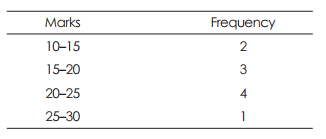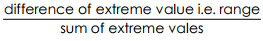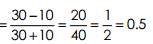Significance & Characteristics - Measures of Dispersion, Business Mathematics & Statistics

# Significance & Characteristics - Measures of Dispersion, Business Mathematics & Statistics - Business Mathematics and Statistics - B Com

MEASURES OF DISPERSION
A measure of dispersion is designed to state the extent to which individual observations (or items) vary from their average. Here we shall account only to the amount of variation (or its degree) and not the direction. Usually, when the deviation of the observations form their average (mean, median or mode) are found out then the average of these deviations is taken to represent a dispersion of a series. This is why measure of dispersion are known as Average of second order. We have seen earlier that mean, median and mode, etc. are all averages of the first order.
Measures of dispersion are mainly of two types– (A) Absolute measures are as follows :
(i) Range,
(ii) Mean deviation (or Average deviation),
(iii) Standard deviation
(B) Among the Relative measures we find the following types :
(i) Coefficient of dispersion.
(ii) Coefficient of variation.

Absolute and Relative measures : If we calculate dispersion of a series, say, marks obtained by students in absolute figures, then dispersion will be also in the same unit (i.e. marks). This is absolute dispersion. If again dispersion is calculated as a ratio (or percentage) of the average, then it is relative dispersion.

RANGE : For a set observations, range is the difference between the extremes, i.e. Range = Maximum value – Minimum value

Illustration 43. The marks obtained by 6 students were 24, 12, 16, 11, . Find the Range. If the highest mark is omitted, find the percentage change in the range.
Here maximum mark = 42, minimum mark = 11.
∴ Range = 42 – 11 = 31 marks
If again the highest mark 42 is omitted, then amongst the remaining. Maximum mark is 40. So, range (revised) = 40 – 11 = 29 marks.
Change in range = 31 – 29 = 2 marks.
∴ Reqd. percentage change = 2 ÷ 31 × 100 = 6.45%
Note: Range and other obsolute measures of dispersion are to be expressed in the same unit in which observations are expressed.

For grouped frequency distribution : In this case range is calculated by subtracting the lower limit of the lowest class interval from the upper limit of the highest.

Example 44 : For the following data calculate range :Here upper limit of the highest class interval = 30 And lower limit of the first class interval = 10
∴ Range = 30 – 10 = 20 marks
Note : Alternative method is to subtract midpoint of the lowest class from that of the highest. In the above case, range = 27.5 – 12.5 = 15 marks.
In practice both the methods are used.

Coefficient of Range :
The formulae of this relative measure isIn the above example, Coefficient of rangeAdvantages of Range : Range is easy to understand and is simple to compute. Disadvantages of Range : It is very much affected by the extreme values. It does not depend on all the observations, but only on the extreme values. Range cannot be computed in case of open-end distribution. Uses of Range : It is popularly used in the field of quality control. In stock-market fluctuations range is used.

Mean Deviation (or Average Deviation):
Mean deviation and standard deviation, however, are computed by taking into account all the observations of the series, unlike range. Definition : Mean deviation of a series is the arithmetic average of the deviations of the various items from the median or mean of that series. Median is preferred since the sum of the deviations from the median is less than from the mean. So the values of mean deviation calculated from median is usually less than that calculated from mean. Mode is not considered, as its value is indeterminate. Mean deviation is known as First Moment of dispersion.

Importance of Dispersion:
We know that the object of measuring dispersion is to ascertain the degree of deviation which exist in the data and hence, the limits within which the data will vary in some measurable variate or attribute or quality. This object of dispersion is of great importance and occupies a unique position in statistical methods.

1. Measures of dispersion supplement the information given by the measures of central tendency:

Measures of dispersion are also called averages of the ‘second order i,e., second time averaging the deviations from a measure of central tendency. It affords an estimate of the phenomena to which the given (original) data relate. This will increase the accuracy of statistical analysis and interpretation and we can be in a position to draw more dependable inferences.

2. Measures of dispersion make possible comparison between different groups:
If the original data is expressed in different units, comparisons will not be possible. But with the help of relative measures of dispersion, all such comparisons can be easily made. Accurate and dependable comparison between the variability of two series will lead to dependable and accurate results.

3. Measures of dispersion are very important in many social problems:
Social problems of different area of the country can be compared with different areas and then these social evils can be removed by taking effective steps.

4. Measures of dispersion serve as a useful check on drawing wrong conclusions from the comparison of averages or measures of central tendency:
The arithmetic mean may be the same of two different groups but it will not reveal about the prosperity of one group and backwardness of other. This type of internal make-up can be known by the study of dispersion.
Thus with the help of the study of dispersion we will not conclude that both the groups are similar. We may find that one group is prosperous and the other is backward by knowing the amount of variability around the measures of central tendency.
Measures of dispersion are of great value in our statistical analysis provided relatives (coefficients of dispersions) are put into practice. Otherwise, conclusions drawn will not be dependable and reliable to a great extent.

The document Significance & Characteristics - Measures of Dispersion, Business Mathematics & Statistics | Business Mathematics and Statistics - B Com is a part of the B Com Course Business Mathematics and Statistics.
All you need of B Com at this link: B Com

115 videos|142 docs

## FAQs on Significance & Characteristics - Measures of Dispersion, Business Mathematics & Statistics - Business Mathematics and Statistics - B Com

 1. What is the significance of measures of dispersion in business mathematics and statistics?Ans. Measures of dispersion in business mathematics and statistics are significant because they provide information about the variability or spread of data. They help in understanding the degree of variation within a dataset, which is crucial for decision-making, risk analysis, and forecasting in business.
 2. What are the characteristics of measures of dispersion?Ans. The characteristics of measures of dispersion include: - They provide an indication of the variability or spread of data. - They are calculated using the original data values and not just their frequencies. - They are affected by extreme values or outliers in the dataset. - They can be measured using various methods such as range, variance, standard deviation, and interquartile range. - They help in comparing the variability between different datasets or groups.
 3. How is range calculated as a measure of dispersion?Ans. The range is a simple measure of dispersion calculated by subtracting the smallest value from the largest value in a dataset. It provides an idea about the spread of data but does not consider the distribution within the range. Range = Largest value - Smallest value.
 4. What is the significance of variance as a measure of dispersion?Ans. Variance is a widely used measure of dispersion that considers the spread of data points around the mean. It provides a more comprehensive understanding of the variability within a dataset compared to the range. Variance is used in statistical analysis, hypothesis testing, and building predictive models in business mathematics and statistics.
 5. How is standard deviation related to variance as a measure of dispersion?Ans. Standard deviation is the square root of the variance and is another commonly used measure of dispersion. It provides a measure of the average distance between each data point and the mean. Standard deviation is preferred over variance in some situations as it is in the same unit as the original data. Both variance and standard deviation indicate the spread of data, but standard deviation is more interpretable and widely used.

115 videos|142 docsExplore Courses for B Com examSignup to see your scores go up within 7 days! Learn & Practice with 1000+ FREE Notes, Videos & Tests.
10M+ students study on EduRev
Track your progress, build streaks, highlight & save important lessons and more!
Related Searches

,

,

,

,

,

,

,

,

,

,

,

,

,

,

,

,

,

,

,

,

,

,

,

,

;